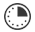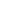# Interest-ing Math

JRDAssist
Middle School and High School Teacher

Students will learn about simple interest and how to calculate the real cost of a loan, credit card, and other types of borrowing.

##Length of Time: 40 - 50 Minutes

Featured Programs:

### Common Core Alignment

CCSS.Math.Content.7.RP.A.3 - Use proportional relationships to solve multistep ratio and percent problems. Examples: simple interest, tax, markups and markdowns, gratuities and commissions, fees, percent increase and decrease, percent error.

## Objectives & Outcomes

The students will be able to use the formula for simple interest, finding the total cost of using various types of loans or credit.

## Materials Needed

• Paper credit cards
• pictures or photos of popular cars/motorcycles with prices (each with different interest rates and terms)
• calculators (optional),
• paper
• pencil

## Procedure

### Opening to Lesson

• Choose several students and give them the choice of taking \$1000 today or waiting one month and taking the money with ten or fifteen percent added to it.
• Ask each of them for their reasons to take it now or wait for the month to pass.
• Introduce the concept of percentage rates and the cost of borrowing money

### Body of Lesson

#### Modeling

• Display the formula I = prt
• “Plug in” the numbers for a new car purchase
• Show an example of purchasing a new car for cash versus paying for it over time
• Explain how the length of the loan affects the total amount the car will end up costing

#### Guided Practice

• Distribute “credit cards” and a “cars” to each student
• The students will choose a credit card and car with the terms they feel would be best for them
• Once they determine the interest, cost of the loan, monthly payments, etc.; allow students to discuss their choices with others
• Ask them if they would change their original choice based on discovering what others are paying
• Discuss the reasons for lower vs. higher interest rates
• Have students list or discuss advantages/disadvantages of taking a longer period of time to pay, paying over time versus paying in cash, etc.

#### Independent Practice

• The teacher will distribute a handout with several similar word problems which will test the students’ skills in determining interest, total cost, monthly payments, etc.
• For homework, “give” students a credit card to use allowing them to make an imaginary purchase costing over \$300.  In essay form, have them explain to another person, who knows nothing about interest, the concept of paying interest on the purchase.
• The teacher will collect the completed page(s).  This will be used as an assessment.

### Closing

Ask students if they were surprised by the cost of purchases with interest added.  Prompt students to discover a method to earn interest such as bank accounts, retirement accounts, etc.  Finally, restate the original question about taking \$1000 today or waiting for a month from now with ten or fifteen percent added.

## Assessment & Evaluation

Handouts/Worksheets with word problems having similar questions related to calculating monthly payments, total cost of loans, etc.

## Modification & Differentiation

Students may work in pairs.  Discuss reasons for different interest rates.  Pros and cons of buying new vs. buying a used product.  Do not use calculators.

## Related Lesson Plans

##### Silent Geometry

The students will practice using geometry formulas measuring items in the classroom to find area and volume, radius and circumference, and identify the types of angles.

##### Numbers By The Book

This lesson will allow students to show a relationship between numbers and a visual representation in a manner that can be used for younger students.

##### Take a Vacation

The students will plan a vacation for themselves, group of friends, or family, considering the costs involved.

##### Box Geometry

Students will learn about using the formulas for the area of squares, rectangles, and triangles to determine how much paint and carpet to purchase for a room.  In addition, they will learn about scale measurements.

##